2019-07-16 13:03:03 ch18328071580 阅读数 279
• ###### 玩转深度学习实战教程

玩转深度学习视频培训课程，详细讲解深度学习的原理和利用深度学习框架TensorFlow进行项目实战。课程通过Kaggle竞赛平台的Titanic问题讲解TensorFlow的基本用法以及问题处理的常用技巧，讲解深度学习图像领域的卷积神经网络CNN和多个经典的网络架构、CNN的应用，讲解自然语言处理领域的RNN、LSTM以及它们的多种变种结构，构建语言模型和对话机器人，介绍损失函数和优化深度学习算法在TensorFlow中的实现。

2981 人正在学习 去看看 王而川

# 一、代价函数概述

• 1.为了得到训练逻辑回归模型的参数，需要一个代价函数，通过训练代价函数来得到参数。
• 2.用于找到最优解的目标函数。

# 二、代价函数的原理

$h(x)=A+Bx$$J(\theta _0,\theta _1)=\frac{1}{2m}\sum_{i=1}^{m}(h(x^{(i)})-y^{(i)})^2$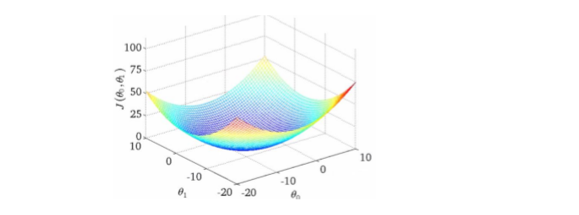• 1.非负性，所以目标就是最小化代价函数。
• 2.当真实输出$a$与期望输出$y$接近的时候，代价函数接近于0。(比如$y=0$$a～0$$y=1$，a~1时，代价函数都接近0)。

• 目标函数存在一个下界，在优化过程当中，如果优化算法能够使目标函数不断减小，根据单调有界准则，这个优化算法就能证明是收敛有效的。
• 只要设计的目标函数有下界，基本上都可以，代价函数非负更为方便。

# 三、常见的代价函数

$J=\frac{1}{2n}\sum_{i=1}\left | \right |y(x)-a^{L}(x)\left | \right |^2$

$J=\frac{(y-a)^2}{2}$

$\frac{\partial J}{\partial w}=(a-y){\delta }'(z)x$
$\frac{\partial J}{\partial b}=(a-y){\delta }'(z)$

## 2. 交叉熵代价函数(cross-entropy)

$J=-\frac{1}{n}\sum_{i=1}[ ylna+(1-y)ln(1-a)]$

$\frac{\partial J}{\partial w_j}=\frac{1}{n}\sum_{x}x_j(\delta(x)-y)$
$\frac{\partial J}{\partial b}=\frac{1}{n}\sum_{x}(\delta(x)-y)$

## 3. 对数似然代价函数(log-likelihood cost)

Softmax回归中将x分类为类别j的概率为：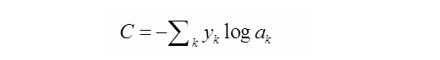# 四、二次代价函数与交叉熵代价函数比较

1.当我们用sigmoid函数作为神经元的激活函数时，最好使用交叉熵代价函数来替代方差代价函数。

• 因为使用二次代价函数时，偏导数受激活函数的导数影响，sigmoid 函数导数在输出接近 0 和 1 时非常小，会导致一 些实例在刚开始训练时学习得非常慢。而使用交叉熵代价函数时，，权重学习的速度受到$(\delta(z)-y)$的影响，更大的误差，就有更快的学习速度，避免了二次代价函数方程中因${\delta }'(z)$
导致的学习缓慢的情况。

2.交叉熵函数的形式是$−[ylna+(1−y)ln(1−a)]$。而不是$−[alny+(1−a)ln(1−y)]$

2017-07-30 21:30:36 u013710265 阅读数 255
• ###### 玩转深度学习实战教程

玩转深度学习视频培训课程，详细讲解深度学习的原理和利用深度学习框架TensorFlow进行项目实战。课程通过Kaggle竞赛平台的Titanic问题讲解TensorFlow的基本用法以及问题处理的常用技巧，讲解深度学习图像领域的卷积神经网络CNN和多个经典的网络架构、CNN的应用，讲解自然语言处理领域的RNN、LSTM以及它们的多种变种结构，构建语言模型和对话机器人，介绍损失函数和优化深度学习算法在TensorFlow中的实现。

2981 人正在学习 去看看 王而川

# 代价函数

## 1. 方差代价函数## 2. 交叉熵代价函数• 非负性。（所以我们的目标就是最小化代价函数）
• 当真实输出a与期望输出y接近的时候，代价函数接近于0.(比如y=0，a～0；y=1，a~1时，代价函数都接近0)。

## 3. 总结

• 当我们用sigmoid函数作为神经元的激活函数时，最好使用交叉熵代价函数来替代方差代价函数，以避免训练过程太慢。
• 不过，你也许会问，为什么是交叉熵函数？导数中不带σ′(z)项的函数有无数种，怎么就想到用交叉熵函数？这自然是有来头的，更深入的讨论就不写了，少年请自行了解。
• 另外，交叉熵函数的形式是−[ylna+(1−y)ln(1−a)]。而不是−[alny+(1−a)ln(1−y)]，为什么？因为当期望输出的y=0时，lny没有意义；当期望y=1时，ln(1-y)没有意义。而因为a是sigmoid函数的实际输出，永远不会等于0或1，只会无限接近于0或者1，因此不存在这个问题。

## 4. log似然代价函数

Softmax回归中将x分类为类别j的概率为：2017-12-01 22:05:47 juyin2015 阅读数 1553
• ###### 玩转深度学习实战教程

玩转深度学习视频培训课程，详细讲解深度学习的原理和利用深度学习框架TensorFlow进行项目实战。课程通过Kaggle竞赛平台的Titanic问题讲解TensorFlow的基本用法以及问题处理的常用技巧，讲解深度学习图像领域的卷积神经网络CNN和多个经典的网络架构、CNN的应用，讲解自然语言处理领域的RNN、LSTM以及它们的多种变种结构，构建语言模型和对话机器人，介绍损失函数和优化深度学习算法在TensorFlow中的实现。

2981 人正在学习 去看看 王而川

Juyin@2017/12/1

2018-01-06 19:27:42 chen645096127 阅读数 4997
• ###### 玩转深度学习实战教程

玩转深度学习视频培训课程，详细讲解深度学习的原理和利用深度学习框架TensorFlow进行项目实战。课程通过Kaggle竞赛平台的Titanic问题讲解TensorFlow的基本用法以及问题处理的常用技巧，讲解深度学习图像领域的卷积神经网络CNN和多个经典的网络架构、CNN的应用，讲解自然语言处理领域的RNN、LSTM以及它们的多种变种结构，构建语言模型和对话机器人，介绍损失函数和优化深度学习算法在TensorFlow中的实现。

2981 人正在学习 去看看 王而川2.交叉熵代价函数(cross-entropy)：3.对数释然代价函数(log-likelihood cost)：

4.总结：

tf.nn.sigmoid_cross_entropy_with_logits()来表示跟sigmoid搭配使用的交叉熵。
tf.nn.softmax_cross_entropy_with_logits()来表示跟softmax搭配使用的交叉熵。

2018-10-11 13:04:21 qq_36890572 阅读数 378
• ###### 玩转深度学习实战教程

玩转深度学习视频培训课程，详细讲解深度学习的原理和利用深度学习框架TensorFlow进行项目实战。课程通过Kaggle竞赛平台的Titanic问题讲解TensorFlow的基本用法以及问题处理的常用技巧，讲解深度学习图像领域的卷积神经网络CNN和多个经典的网络架构、CNN的应用，讲解自然语言处理领域的RNN、LSTM以及它们的多种变种结构，构建语言模型和对话机器人，介绍损失函数和优化深度学习算法在TensorFlow中的实现。

2981 人正在学习 去看看 王而川

1.代价函数

1.1 代价函数：任何可以衡量模型预测结果值与其真实值之间差异的函数，如果存在多个样本，代价函数统计结果应该为所有样本的差异之和；

1.2 代价函数即模型误差，是反向传播中求梯度的基础，同时也是模型最终达到期望效果的衡量指标；

1.3 主要的代价函数

a. 二次代价函数(均方误差)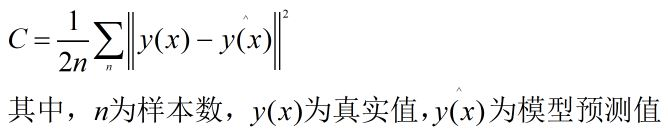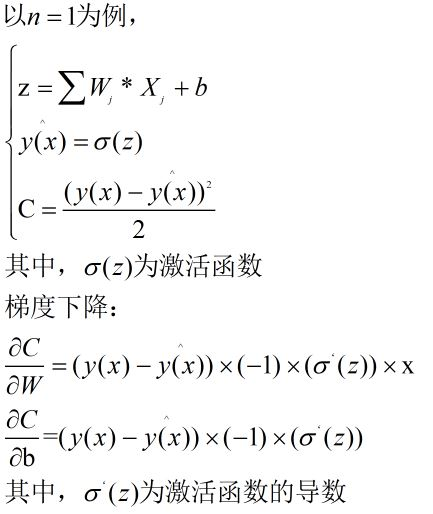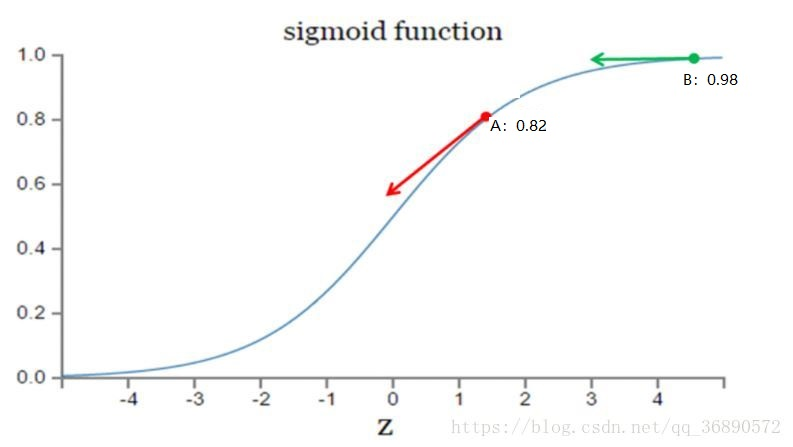b. 交叉熵代价函数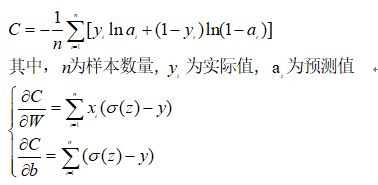c. 对数似然函数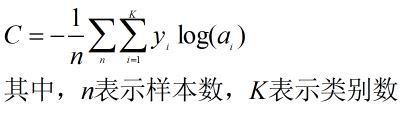# 在手写数字识别中
# 对于出现概率最大的数字与真实值之间求softmax分类概率，对分类概率求交叉熵，最后求其平均值
ce = tf.reduce_mean(tf.nn.sparse_softmax_cross_entropy_with_logits(logits=y,labels=tf.arg_max(y_predict,1)))

1.4 常见模型的代价函数

a. 逻辑回归 ：交叉熵

b. 线性回归：均方误差

c. 多分类问题(softmax)：对数似然